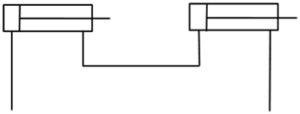# 3.1: Series and Parallel Hydraulic Circuits

$$\newcommand{\vecs}{\overset { \rightharpoonup} {\mathbf{#1}} }$$ $$\newcommand{\vecd}{\overset{-\!-\!\rightharpoonup}{\vphantom{a}\smash {#1}}}$$$$\newcommand{\id}{\mathrm{id}}$$ $$\newcommand{\Span}{\mathrm{span}}$$ $$\newcommand{\kernel}{\mathrm{null}\,}$$ $$\newcommand{\range}{\mathrm{range}\,}$$ $$\newcommand{\RealPart}{\mathrm{Re}}$$ $$\newcommand{\ImaginaryPart}{\mathrm{Im}}$$ $$\newcommand{\Argument}{\mathrm{Arg}}$$ $$\newcommand{\norm}{\| #1 \|}$$ $$\newcommand{\inner}{\langle #1, #2 \rangle}$$ $$\newcommand{\Span}{\mathrm{span}}$$ $$\newcommand{\id}{\mathrm{id}}$$ $$\newcommand{\Span}{\mathrm{span}}$$ $$\newcommand{\kernel}{\mathrm{null}\,}$$ $$\newcommand{\range}{\mathrm{range}\,}$$ $$\newcommand{\RealPart}{\mathrm{Re}}$$ $$\newcommand{\ImaginaryPart}{\mathrm{Im}}$$ $$\newcommand{\Argument}{\mathrm{Arg}}$$ $$\newcommand{\norm}{\| #1 \|}$$ $$\newcommand{\inner}{\langle #1, #2 \rangle}$$ $$\newcommand{\Span}{\mathrm{span}}$$$$\newcommand{\AA}{\unicode[.8,0]{x212B}}$$A YouTube element has been excluded from this version of the text. You can view it online here: https://openoregon.pressbooks.pub/hydraulics/?p=72

Describe flow paths in basic series hydraulic circuits

Describe the actuation sequence of actuators in a series relationship.

Discuss the source of differences in travel length and speed for series hydraulic circuits.Given two cylinders with the following dimensions in a series relationship, calculate the extension distance of the downstream cylinder given the upstream cylinder fully extends

cap = 1 3/4”

rod = 5/8

travel = 6”

Given the dimensions of the upstream cylinder are to remain fixed, determine the diameter of the downstream cylinder such that it fully extends 6” when the upstream cylinder reaches the limits of travel.

Given two cylinders with the following dimensions in a series relationship, calculate the maximum extension force the system is capable of exerting with the downstream cylinder given input to the upstream cylinder’s cap in limited to 490psi.

cap = 1 3/4”

rod = 5/8

travel = 6”

Given the downstream cylinder is loaded with an 800lbf object determine the pressure in the cap end of the downstream cylinder, the force exerted by the upstream cylinder, and the pressure in the cap end of the upstream cylinder.

Comment on advantages and disadvantages of pressure intensification for series hydraulic circuits.

Given an intensifier with the following dimensions, calculate the output pressure given an input pressure of 80psi.

din = 3”

dout= 3/4”

Describe flow paths in basic parallel hydraulic circuits.Given two cylinders in a parallel relationship with the following dimensions determine the extension sequence if cylinder A is loaded with a 500lbf load and cylinder B is loaded with a 600lbf load. Calculate the extension pressure necessary to move A. Calculate the extension pressure necessary to move B.

cap = 1 3/4”

rod = 5/8

travel = 6”

Given two cylinders in a parallel relationship with the following dimensions calculate the extension sequence if the cylinders are both loaded with 800lbf loads. Calculate the extension pressure necessary to move A. Calculate the extension pressure necessary to move B.

A cap = 1 3/4”

A rod = 5/8

A travel = 6”

B cap = 2”

B rod = 5/8

B travel = 6”

Discuss the purpose of a mechanical yoke, balanced loads, and flow control valves in a parallel relationship.

Given two cylinders in a parallel relationship with the following dimensions mechanically yoked together calculate the extension pressure necessary to move a perfectly balanced 960lbf object.

cap = 1 3/4”

rod = 5/8

travel = 6”

This page titled 3.1: Series and Parallel Hydraulic Circuits is shared under a CC BY-NC 4.0 license and was authored, remixed, and/or curated by Jim Pytel (OpenOregon) via source content that was edited to the style and standards of the LibreTexts platform; a detailed edit history is available upon request.